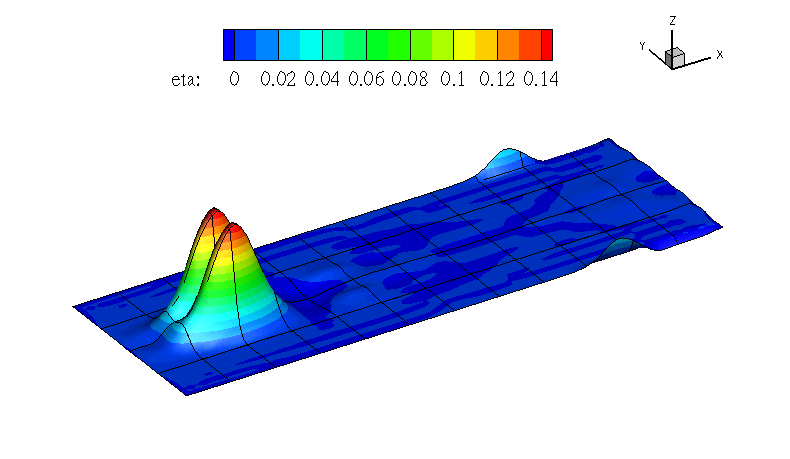### 14.4 Examples

#### 14.4.1 Rossby modon case

This example, provided in `RossbyModon_Nonlinear_DG.xml` is of a discontinuous Galerkin simulation of the westward propagation of an equatorial Rossby modon.

##### 14.4.1.1 Input Options

For what concern the ShallowWaterSolver the `<TIMEINTEGRATIONSCHEME>` and `<SOLVERINFO>` section allows us to specify the solver, the type of projection (continuous or discontinuous), the explicit time integration scheme to use and (in the case the discontinuous Galerkin method is used) the choice of numerical flux. A typical example would be:

1 <TIMEINTEGRATIONSCHEME>
2    <METHOD> RungeKutta </METHOD>
3    <ORDER> 4 </ORDER>
4 </TIMEINTEGRATIONSCHEME>
1 <SOLVERINFO>
2   <I PROPERTY="EqType"                VALUE="NonlinearSWE"         />
3   <I PROPERTY="Projection"            VALUE="DisContinuous"        />
4   <I PROPERTY="UpwindType"            VALUE="HLLC"                 />
5 </SOLVERINFO>

In the `<PARAMETERS>` section we, in addition to the normal setting of time step etc., also define the acceleration of gravity by setting the parameter "Gravity":

1  <PARAMETERS>
2     <P> TimeStep       = 0.04             </P>
3     <P> NumSteps       = 1000             </P>
4     <P> IO_CheckSteps  = 100              </P>
5     <P> IO_InfoSteps   = 100              </P>
6     <P> Gravity        = 1.0              </P>
7  </PARAMETERS>

We specify f which is the Coriolis parameter and d denoting the still water depth as analytic functions:

1<FUNCTION NAME="Coriolis">
2    <E VAR="f" VALUE="0+1*y" />
3</FUNCTION>
4
5<FUNCTION NAME="WaterDepth">
6    <E VAR="d" VALUE="1" />
7</FUNCTION>

Initial values and boundary conditions are given in terms of primitive variables (please note that also the output files are given in terms of primitive variables). For the discontinuous Galerkin we typically enforce any slip wall boundaries weakly using symmetry technique. This is given by the `USERDEFINEDTYPE="Wall"` choice in the `<BOUNDARYCONDITIONS>` section:

1  <BOUNDARYCONDITIONS>
2    <REGION REF="0">
3     <D VAR="eta"  USERDEFINEDTYPE="Wall"  VALUE="0" />
4     <D VAR="u"    USERDEFINEDTYPE="Wall"  VALUE="0" />
5     <D VAR="v"    USERDEFINEDTYPE="Wall"  VALUE="0" />
6    </REGION>
7  </BOUNDARYCONDITIONS>

##### 14.4.1.2 Running the code

After the input file has been copied to the build directory of the `ShallowWaterSolver` the code can be executed by:

./ShallowWaterSolver Rossby_Nonlinear_DG.xml

##### 14.4.1.3 Post-proceesing

After the final time step the solver will write an output file `RossbyModon_Nonlinear_DG.fld`. We can convert it to tecplot format by using the `FieldConvert` utility. Thus we execute the following command:

FieldConvert RossbyModon_Nonlinear_DG.xml RossbyModon_Nonlinear_DG.fld \
RossbyModon_Nonlinear_DG.dat

This will generate a file called `RossbyModon_Nonlinear_DG.dat` that can be loaded directly into tecplot: